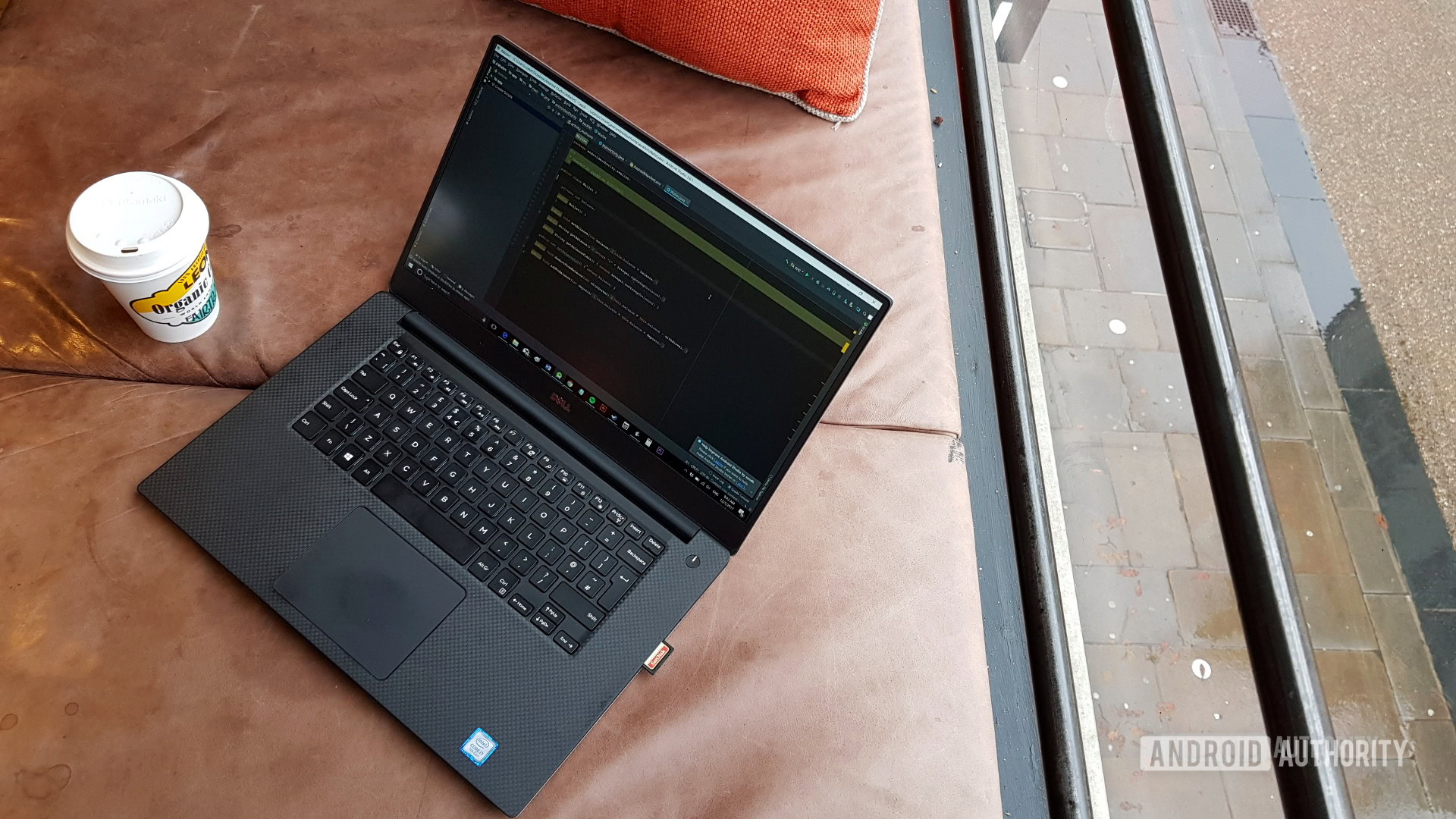Best daily deals

# How to use if statements in Python

This post explains how to use if statements in Python
By
February 21, 2021If statements are among the first things you should learn in any programming language, and are required for pretty much any useful code. In this post, we’ll take a look at how to use if statements in Python, so that you can begin building useful apps!

Once you understand this fundamental feature, you’ll open up a whole world of possibilities!

## How to use if statements in Python

If you have never programmed before, then make sure to read the next section to find out precisely what an “if statement” is, and how to use it.

Also read: How to call a function in Python

If you have coding experience and you just want to know how to use if statements in Python, then read on:

Code
``````if magic_number == 7:
print("The number is correct!")``````

Simply follow the word “if” with the statement you want to test, and then add a colon. The following code block (all indented text) will run only if the statement is true.

## What are if statements in Python?

For those with no programming experience, an “if statement” is a piece of code that is used for “flow control.” This means that you’ve created a kind of fork in the road: a point in your program where the flow of events can branch off into two or more paths.

This is essential in any program, as it is what allows a program to interact with the user, or to change dynamically in response to outside factors.

Also read: How to use lists in Python

The “if statement” in Python does this specifically by testing whether a statement is true, and then executing a code block only if it is.

In other words:

“IF this is true, THEN do this.”

In a program, this might translate to:

“IF the user enters the correct password, THEN grant access.”

“IF the player has 0 health, THEN end the game.”

Now the code can react depending on various factors and inputs, creating an interactive experience for the user!

In order to accomplish this, we must rely on one more advanced concept: the variable. A variable is a word that represents a piece of data. For example, we can say:

Code
``magic_number = 7``

This creates a variable called “magic_number” and gives it the value of seven. This is important, because we can now test if that value is correct.

To do this, we write “if” and then the statement we want to test. This is called the “test statement.”

When checking the value of something, we use two equals signs. While this might seem confusing, this actually avoids confusion; we only use a single equals sign when we are assigning value.

After the statement, we add a colon, and then an indentation. All code that is indented after this point belongs to the same “code block” and will only run if the value is true.

Code
``````magic_number = 7

if magic_number == 7:
print("The number is correct!")

print("Did you get it right?")``````

In this example, the words “Did you get it right?” will show whatever the case. But if you change the value of magic_number to “8” then you won’t see “The number is correct!” on the screen.

## How to use if statements in Python with else

Finally, you may also want to combine if statements with “else” statements. Else does exactly what it sounds like: it tells Python what to do if the value isn’t true.

For example, we might want to check someone’s PIN number:

Code
``````pin_number = 7321

if pin_number == 7321:
print("Correct pin!")
else:
print("Incorrect pin!")

print(“Did you get it right?”)``````

Here, the “else” code only runs if the PIN is incorrect. “Did you get it right?” still shows no matter what happens!

We can also use a similar variation called “else if” or “elif.” This means “if that thing isn’t true, but this other thing is.”

For example:

Code
``````jeffs_pin = 7321
bobs_pin = 2212
enterred_pin = 7321

if enterred_pin == jeffs_pin:
print("Welcome Jeff!")
elif enterred_pin == bobs_pin:
print("Welcome Bob!")
else:
print("Incorrect PIN")

print("What would you like to do?")``````

Notice that this example also compares two different variables with one another!

## More tricks

Now you know the basics of how to use if statements in Python, but there are many more things you can do.

For example, you can use different “operators” to create different test-statements. For instance, the “>” symbol means bigger than, while “<” means smaller than.

Thus, we can say: if “health” is smaller than one, then gameover.

It’s also possible to “nest” ifs and elses by indenting more and more. This way, you can say “if this is true then do this but only if that is ALSO true.”

Similarly, we can use statements called “and” and “or” in order to add multiple test statements.

For example:

Code
``````if enterred_pin == jeffs_pin and username == "Jeff":
print("Welcome Jeff!")``````

Or:

Code
``````if enterred_pin == jeffs_pin or enterred_pin == bobs_pin:
print("Welcome!")``````

Now you understand how to use if statements in Python, you have a crucial tool under your belt! This will form the backbone of much of your programming, and will help you to run all kinds of logic tests.

So why not take your knowledge further with an online Pythohn course? You can find a list of our favorites to get started with here.

Or, for a more in-depth tutorial right here that explains everything you need to know to start coding in Python, check out our comprehensive Python beginners’ guide.

For more developer news, features, and tutorials from Android Authority, don’t miss signing up for the monthly newsletter below!